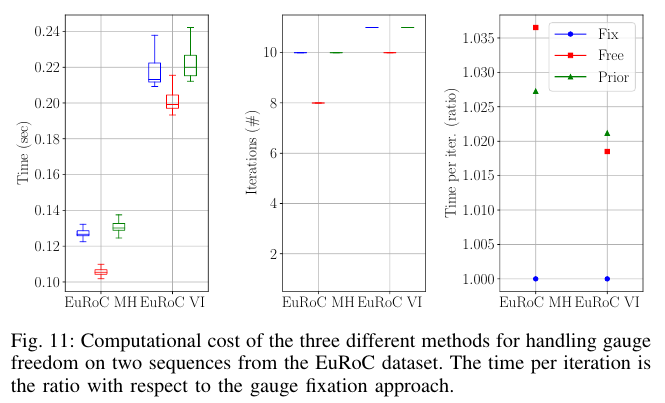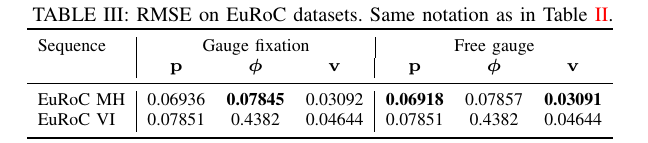# 1. On the Comparison of Gauge Freedom Handling in Optimization-based Visual-Inertial State Estimation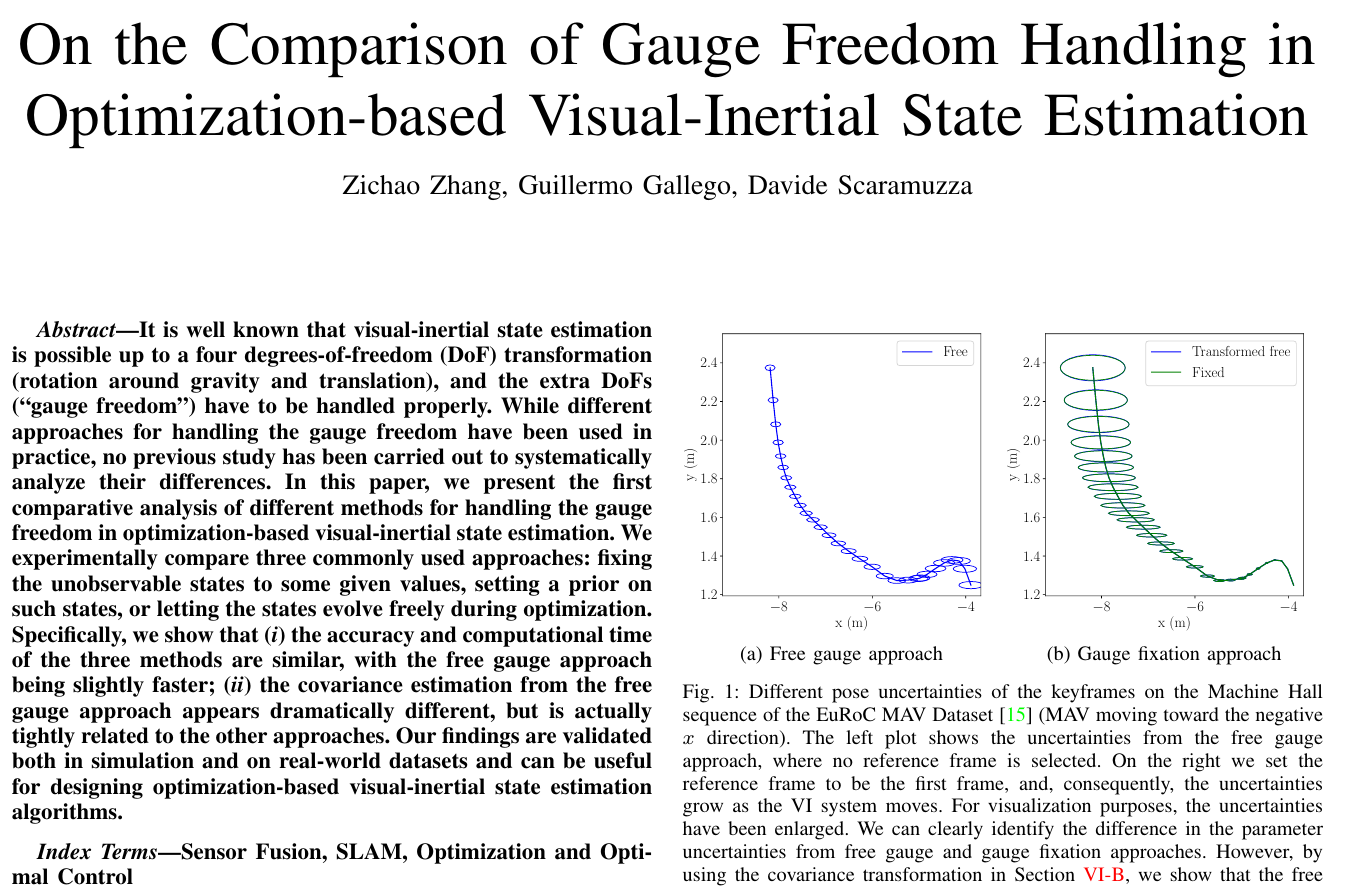# 2. 摘要

VI系统中有4个不可观测自由度，绕重力方向的旋转和平移(在本文中称为$$gauge~freedom$$)，以及剩下一些可观的自由度，在求解过程中需要进行特殊的处理。 文章对比了以下3种处理方法:

• gauge fixation: 设置不可观测的状态为某个固定的值
• gauge prior: 为某个状态设置先验
• free gauge: 让状态在优化过程中自由演化，优化完成再进行处理

# 3. VI问题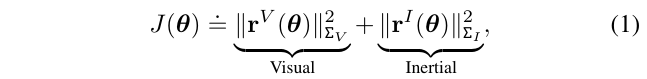• $$||r||_\Sigma^2=r^T\Sigma^{-1}r$$是残差向量$$r$$的马氏距离
• $$\Sigma$$是测量值的协方差矩阵，作为权重
• 这个cost func 可以作为 "full smoothing"或者"fixed-lag smooth"方法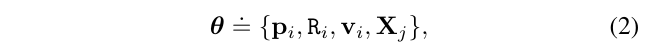## 3.1. 解的歧义性和几何等价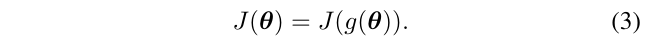• $$t \in \R^3$$是任意的平移
• 旋转$$R_z=\exp (\alpha e_z)$$是绕重力方向轴$$e_z=(0,0,1)^T$$的任意yaw角$$\alpha \in (-\pi,\pi)$$所产生的旋转矩阵
• 为了简化表达，使用$$Exp(\theta)=\exp(\theta^\wedge)$$来表示这个变换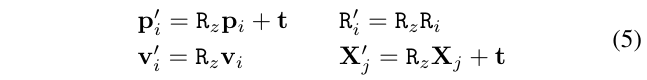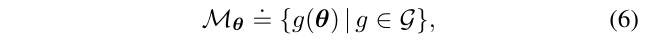• $$\mathcal{G}$$$$g()$$变换的群，注意到目标函数在每一个$$orbit$$上都是常数(意思是存在变换$$g()$$，都不会改变目标函数的值)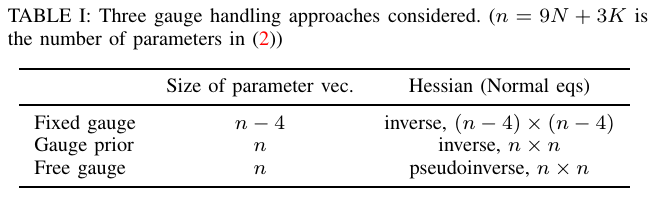• 总的参数$$n=9N+3K$$，N是相机节点数，K是路标数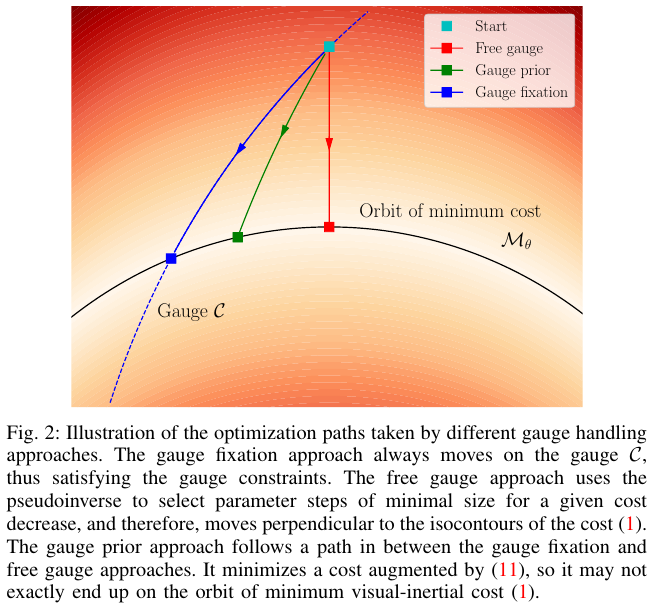## 3.2. 附加约束:指定一个Gauge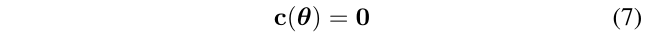$\theta_C=\mathcal{C}\cap \mathcal{M}_\theta$

# 4. 处理Gauge

• (gauge fixation approach)是在一个较小的参数空间中进行优化，在这个空间中没有不可观测的状态，因此Hessian是可逆的，这本质上强制了解的硬约束
• (gauge prior approach)是通过附加惩罚(来产生一个可逆的Hessian)来增强目标函数，以使解决方案以一种软的方式满足某些约束
• (free gauge approach)使用H的伪逆，来隐式地为唯一解决方案提供额外的约束(用最小范数更新参数)

## 4.1. Rotation Parametrization for Gauge Fixation or Prior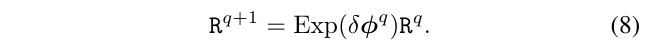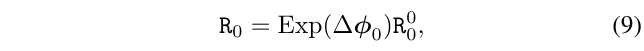## 4.2. 处理Gauge Freedom的不同方法

### 4.2.1. gauge fixation approach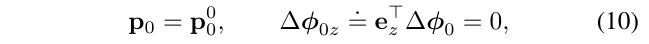### 4.2.2. gauge prior approach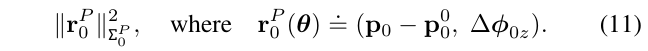# 5. 三种处理方式的对比流程

## 5.1. 数据生成

• 平面的，3D点粗略的分布在几个平面上
• 随机的，3D点随机沿着轨迹生成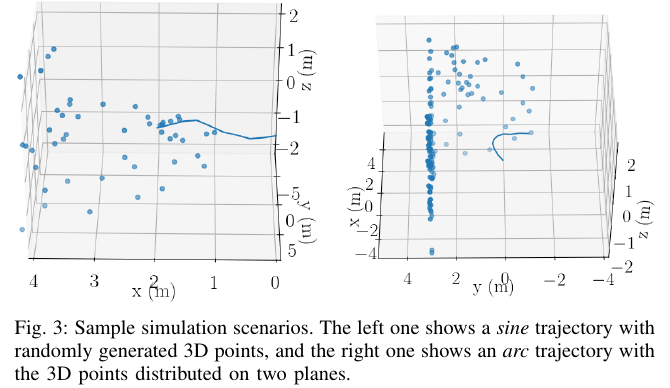## 5.3. 评估

1. 准确率:

为了评估状态估计值的准确率，我们首先计算了将估计值对齐到真值的变换，这个变换从所有轨迹的第一帧开始计算，这个变换具有4自由度(也就是上面提到的平移和绕重力方向矢量的旋转)。

对齐之后，我们计算所有关键帧的均方差根(RMSE)，特别的，我们对位置和速度误差使用欧几里得距离来计算。

对于旋转估计值，我们首先计算估计值和真值的相对旋转量(轴角形式表示)，然后使用相对旋转的角度作为旋转误差。

2. 计算效率:

为了评估算力消耗，我们记录了求解器的收敛的时间和迭代次数。我们使用每种配置(如轨迹和3D点的结合)，进行50次试验，计算平均时间和精度指标

3. 协方差:

我们同样比较由优化算法产生的协方差，这些协方差对active SLAM等应用有重要意义。

参数估计值的协方差由Hessian矩阵的逆确定。(对于free gauge approach，使用摩尔-彭罗斯伪逆，因为Hessian是奇异的)

# 6. 对比结果研究

## 6.1. Gauge Prior: 选择恰当的先验权重

### 6.1.1. Accuracy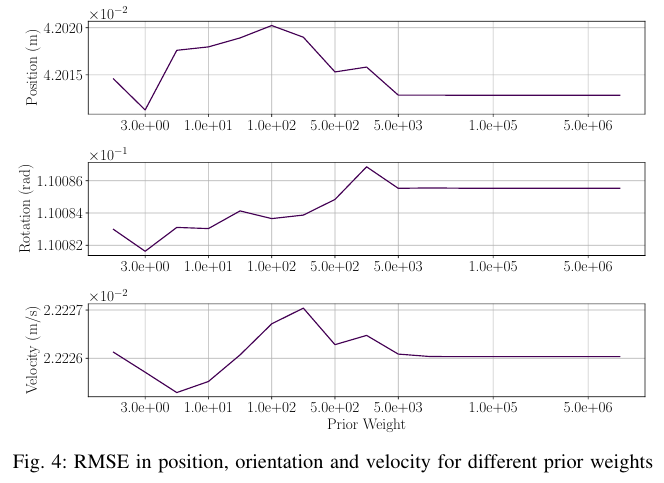### 6.1.2. Computational Cost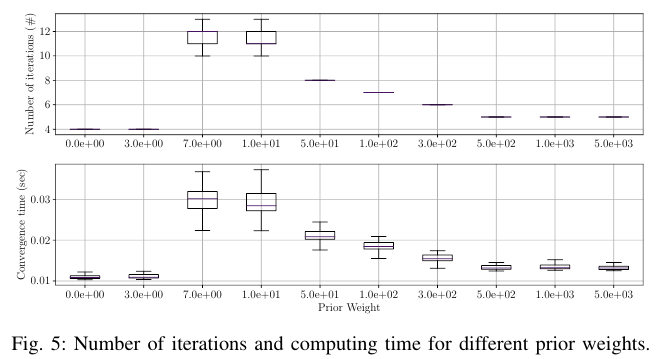• 位置先验误差是第一个位置的当前估计值与其初始值之间的欧氏距离
• 偏航先验误差是第一个旋转的当前估计相对于其初始值的相对旋转的z分量
• 平均重投影误差为所有关键帧中观察到的三维点的个数所平均得到的总视觉残差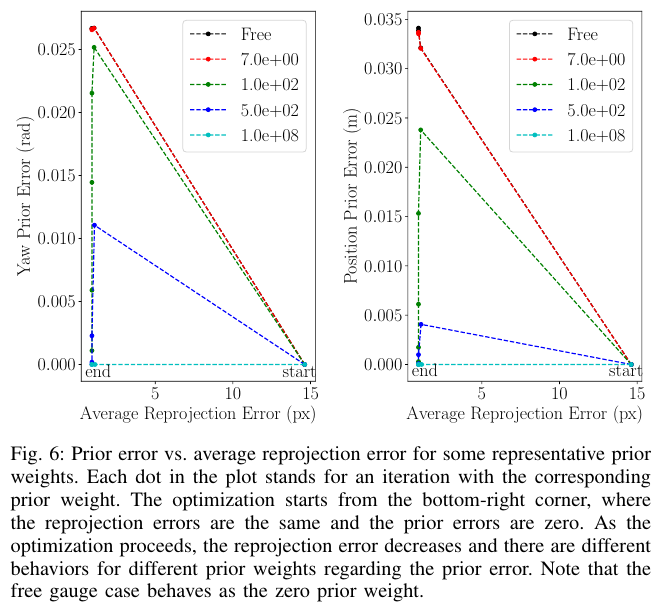## 6.2. 准确度和计算量

• 真值位置使用5cm的向量进行扰动(对于整条轨迹就是5m)
• 旋转量使用随机旋转6度进行扰动
• 速度使用均匀分布随机扰动[-0.05,0.05]m/s ，(速度均值是2m/s)
• 3D点的位置采用均匀随机变量[-7.5,7.5]cm来进行扰动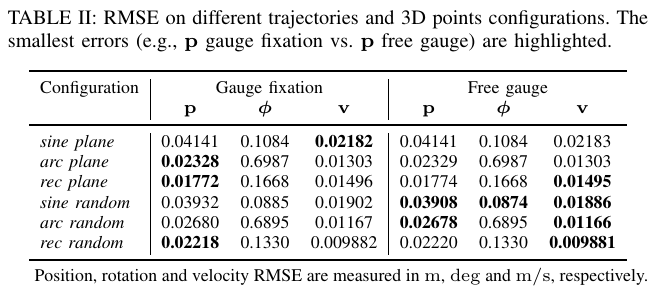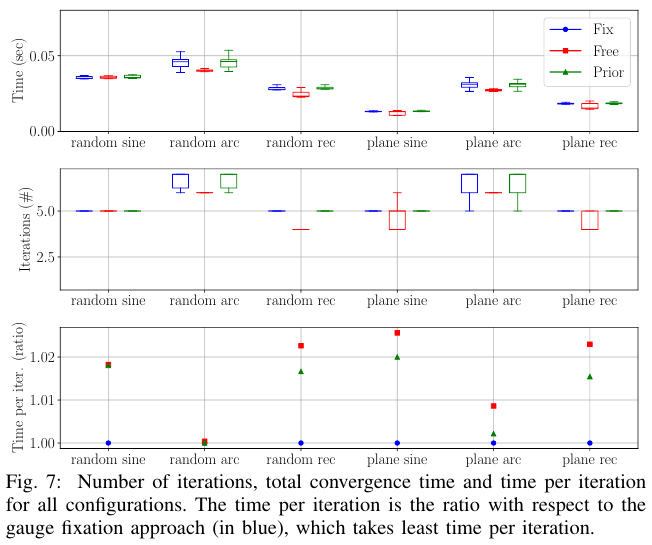gauge prior approachgauge fixation approach的计算量大体相同，而第三种方法即free gauge approach比前两者稍微更快一些。

## 6.3. 小结

• 这三种方法的精确度几乎相同
• gauge prior approach，需要选择合适的先验权重来避免计算量的增大
• 如果选择合适的先验权重，那么gauge prior approach将会与gauge fixation approach具有几乎一致的性能表现(精确度和计算量)
• free gauge approach比其他两种方法稍微快一些，因为它只需更少的迭代次数即可收敛

# 7. 真实世界数据集测试